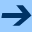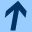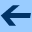Testing with Junit

# Testing with Junit

We have talked before about incremental testing of our functions. We will now create more systematic tests for our objects and methods.

To use JUnit, you must have installed it as explained in the installation instructions.

JUnit is a Java library that allows us to write test suites. A test suite is a class that contains an arbitrary number of tests, checking the correctness of our code.

#### A simple test suite

Let's start with a simple example (test1.kt):

import org.junit.Assert.*
import org.junit.Test

class AdditionTest {

@Test
fun onePlusOne() {
assertEquals("1 + 1 must be 2", 2, 1 + 1)
assertNotEquals("1 + 1 must not be 3", 3, 1 + 1)
}
}


We compile this class:

$ktc test1.kt  If you now check the contents of the classes directory, you'll find a new file AdditionTest.class there. We can run the test suite as follows: $ kttest AdditionTest
JUnit version 4.12
.
Time: 0.004

OK (1 test)


The output shows that everything went alright, all tests were passed.

To demonstrate what it looks like when tests fail, I will change the second assertion in the test like this:

  assertNotEquals("1 + 1 must not be 2", 2, 1 + 1)


Again I compile and run the test:

$ktc test1.kt$ kttest AdditionTest
JUnit version 4.12
.E
Time: 0.005
There was 1 failure:
1) onePlusOne(AdditionTest)
java.lang.AssertionError: 1 + 1 must not be 2. Actual: 2
at org.junit.Assert.fail(Assert.java:88)


#### Checking that exceptions are thrown

To check that our code correctly handles input where we need to throw an exception, we need to write tests where we indicate that an exception is actually expected. This is done using an annotation on the test (test2.kt):

import org.junit.Assert.*
import org.junit.Test

class ArithmeticTest {

@Test
fun onePlusOne() {
assertEquals("1 + 1 must be 2", 2, 1 + 1)
assertNotEquals("1 + 1 must not be 3", 3, 1 + 1)
}

@Test(expected = ArithmeticException::class)
fun divisionByZero() {
@Suppress("UNUSED_VARIABLE")
val result = 1 / 0
}
}



Here is the output:

$ktc test2.kt test2.kt:14:18: warning: division by zero$ kttest ArithmeticTest
JUnit version 4.12
..
Time: 0.004

OK (2 tests)


If we change 1/0 to 1/1, so that no exception will be thrown, the test suite correctly detects the error:

$kttest ArithmeticTest JUnit version 4.12 ..E Time: 0.006 There was 1 failure: 1) divisionByZero(ArithmeticTest) java.lang.AssertionError: Expected exception: java.lang.ArithmeticException at org.junit.internal.runners.statements.ExpectException.evaluate(ExpectException.java:32)  #### Testing our own classes For the polynomial calculator project, I have written a class Polynomial implemented in polynomial.kt. We will now write a test suite for the Polynomial class. This is much better than testing it interactively, because when a test fails, you can modify your code and run all tests again. If you ever need to add features to your classes or change them in any way, it is very useful to have access to all the tests you used previously: You can run them again to make sure that you have not broken anything. It is a good habit to create test suites for all interesting classes that your write. You can use the test suite to have confidence that the class you implemented is correct. And even more useful, it gives you confidence that when you make a change, you do not break some part of the class by mistake. Here is a test suite for the Polynomial class. Note that it simply uses the Polynomial class for its tests (polytest.kt): import org.junit.Assert.* import org.junit.Test class PolynomialTest { @Test fun creatingPolynomials() { val p1 = Polynomial(3) assertEquals(p1.degree(), 0) assertEquals(p1.toString(), "3") val p2 = Polynomial(-1, 3, -4, 0, -6) assertEquals(p2.degree(), 4) assertEquals(p2.toString(), "-6 * X^4 - 4 * X^2 + 3 * X - 1") val p3 = Polynomial(0, 0, 1) assertEquals(p3.degree(), 2) assertEquals(p3.toString(), "X^2") val p0 = Polynomial(0) assertEquals(p0.degree(), -1) } @Test fun additionAndSubtraction() { val p1 = Polynomial(3) val p2 = Polynomial(-1, 3, -4, 0, -6) val p3 = Polynomial(5, 0, 4, 0, -6) val q1 = p1 + p2 val q2 = p2 - p3 assertEquals(q1.toString(), "-6 * X^4 - 4 * X^2 + 3 * X + 2") assertEquals(q2.degree(), 2) assertEquals(q2.toString(), "-8 * X^2 + 3 * X - 6") } @Test fun multiplication() { val p1 = Polynomial(3) val p2 = Polynomial(-1, 3, -4, 0, -6) val p3 = Polynomial(0, 0, 5) val p4 = Polynomial(2, -4, 6, 8) val q1 = p1 * p2 val q4 = p2 * p3 val q5 = p2 * p4 assertEquals(q1.toString(), "-18 * X^4 - 12 * X^2 + 9 * X - 3") assertEquals(q4.degree(), 6) assertEquals(q4.toString(), "-30 * X^6 - 20 * X^4 + 15 * X^3 - 5 * X^2") assertEquals(q5.toString(), "-48 * X^7 - 36 * X^6 - 8 * X^5 - 12 * X^4 + 26 * X^3 - 26 * X^2 + 10 * X - 2") } @Test fun power() { val p1 = Polynomial(3) val p3 = Polynomial(0, 0, 5) val q2 = p1 pow 5 val q3 = p3 pow 5 assertEquals(q2.degree(), 0) assertEquals(q2.coeff(0), 3*3*3*3*3) assertEquals(q3.degree(), 10) assertEquals(q3.toString(), "3125 * X^10") } @Test fun creatingPolynomialsUsingX() { assertEquals(X.toString(), "X") val p4 = -1 * (X pow 5) + 3 * (X pow 3) - (X pow 2) + 5 assertEquals(p4.toString(), "-X^5 + 3 * X^3 - X^2 + 5") val p5 = (X - 1) * (X - 3) * (X + 5) pow 2 assertEquals(p5.toString(), "X^6 + 2 * X^5 - 33 * X^4 - 4 * X^3 + 319 * X^2 - 510 * X + 225") } @Test fun evaluation() { val p1 = Polynomial(3) val p2 = Polynomial(-1, 3, -4, 0, -6) val p3 = Polynomial(0, 0, 1) val p4 = -1 * (X pow 5) + 3 * (X pow 3) - (X pow 2) + 5 val p5 = (X - 1) * (X - 3) * (X + 5) pow 2 val eps = 1.0e-9 // floating point precision assertEquals(3.0, p1(5.0), eps) assertEquals(-1.0, p2(0.0), eps) assertEquals(4.0, p3(2.0), eps) assertEquals(2.0, p4(-1.0), eps) assertEquals(0.0, p5(-5.0), eps) } @Test fun derivatives() { @Suppress("UNUSED_VARIABLE") val p1 = (X - 1) * (X - 3) * ((X + 5) pow 2) /* val q1 = p1.derivative() assertEquals(q1.degree(), 3) assertEquals(q1.toString(), "4 * X^3 + 18 * X^2 - 24 * X - 70") val q2 = q1.derivative() assertEquals(q2.degree(), 2) assertEquals(q2.toString(), "12 * X^2 + 36 * X - 24") */ } }  To run the test suite, we of course first have to compile the Polynomial class, then the PolynomialTest class, and finally we can run the test suite: $ ktc polynomial.kt
$ktc polytest.kt$ kttest PolynomialTest
JUnit version 4.12
.......
Time: 0.03

OK (7 tests)


When you make changes to the Polynomial class, you can run the test suite after each change to make sure everything is still working.

#### Starting with the test suite

We can take this one step further. We can think about a test suite as an executable specification of the correct behavior of the class that we want to create. So instead of writing code first and later testing it using the test suite, we first write down a list of tests that our code must satisfy to be correct. Then we start implementing the methods of the object one by one. After each change, a few more tests will pass correctly. When all tests are passed, we are done with the implementation.

Let's go back to our Polynomial object and assume that it is not already given to us: We should implement it from scratch.

This time we start with the test suite polytest.kt. Here we immediately encounter a problem: Since PolynomialTest uses the Polynomial object, it is impossible to compile polytest.kt without a Polynomial class.

So what we will do is to create an empty framework for the Polynomial class that shows all the methods and their types. Using this framework, we can not only compile the test suite, but we now also have a full documentation of the methods that we plan to implement.

So the first version of my Polynomial class looks like this (polynomial1.kt):

@Suppress("UNUSED_PARAMETER")
class Polynomial(coeffs: Array<Int>) {

constructor(vararg coeffs: Int) : this(coeffs.toTypedArray()) { }

fun degree(): Int = TODO()

fun coeff(i: Int): Int = TODO()

override fun toString(): String = TODO()

operator fun plus (rhs: Polynomial): Polynomial = TODO()

operator fun plus(rhs: Int) = this + Polynomial(rhs)

operator fun minus (rhs: Polynomial): Polynomial = TODO()

operator fun minus(rhs: Int) = this - Polynomial(rhs)

operator fun times (rhs: Polynomial): Polynomial = TODO()

infix fun pow (ex: Int): Polynomial = TODO()

operator fun invoke(x: Double): Double = TODO()
}

operator fun Int.times(rhs: Polynomial) = Polynomial(this) * rhs

val X = Polynomial(0, 1)


I've defined all the public methods of the class that I plan to implement, including the types of arguments and the result type. Each method is defined to return TODO(). This code can already be compiled:

$ktc polynomial1.kt  And now we can compile the test suite without any errors: $ ktc polytest.kt


We run our test suite:

$kttest PolynomialTest JUnit version 4.12 .E.E.E.E.E.E.E Time: 0.026 There were 7 failures: 1) creatingPolynomials(PolynomialTest) kotlin.NotImplementedError: An operation is not implemented. at Polynomial.degree(polynomial1.kt:31) 2) power(PolynomialTest) kotlin.NotImplementedError: An operation is not implemented. at Polynomial.pow(polynomial1.kt:31) 3) additionAndSubtraction(PolynomialTest) kotlin.NotImplementedError: An operation is not implemented. at Polynomial.plus(polynomial1.kt:31) 4) multiplication(PolynomialTest) kotlin.NotImplementedError: An operation is not implemented. at Polynomial.times(polynomial1.kt:31) 5) evaluation(PolynomialTest) kotlin.NotImplementedError: An operation is not implemented. at Polynomial.pow(polynomial1.kt:31) 6) creatingPolynomialsUsingX(PolynomialTest) kotlin.NotImplementedError: An operation is not implemented. at Polynomial.toString(polynomial1.kt:31) 7) derivatives(PolynomialTest) kotlin.NotImplementedError: An operation is not implemented. at Polynomial.minus(polynomial1.kt:31) FAILURES!!! Tests run: 7, Failures: 7  Of course all tests fail, because our class does not do anything yet. In particular, if you try to call the function TODO, it raises a NotImplementedError. (TODO is a special function with result type Nothing. Since Nothing is a subtype of every Kotlin type, it is legal to write TODO() instead of any type. However, there is no object of type Nothing, and so the function must throw an exception.) Now we are set up to build our Polynomial class. I start by adding a constructor, implement degree and coeff, and write a toString method (polynomial2.kt): @Suppress("UNUSED_PARAMETER") class Polynomial(coeffs: Array<Int>) { constructor(vararg coeffs: Int) : this(coeffs.toTypedArray()) { } private val c = createCoeffs(coeffs) private fun createCoeffs(a: Array<Int>): List<Int> { var s = a.lastIndex while (s >= 0 && a[s] == 0) s -= 1 return a.take(s+1) } fun degree(): Int = c.lastIndex fun coeff(i: Int): Int = if (i < c.size) c[i] else 0 override fun toString(): String { var s = StringBuilder() var plus = "" var minus = "-" for (i in degree() downTo 0) { if (c[i] != 0) { var e = c[i] s.append(if (e > 0) plus else minus) plus = " + "; minus = " - " e = Math.abs(e) if (i == 0) s.append(e) else { if (e != 1) { s.append(e) s.append(" * ") } if (i > 1) { s.append("X^") s.append(i) } else s.append("X") } } } return s.toString() } operator fun plus (rhs: Polynomial): Polynomial = TODO() operator fun plus(rhs: Int) = this + Polynomial(rhs) operator fun minus (rhs: Polynomial): Polynomial = TODO() operator fun minus(rhs: Int) = this - Polynomial(rhs) operator fun times (rhs: Polynomial): Polynomial = TODO() infix fun pow (ex: Int): Polynomial = TODO() operator fun invoke(x: Double): Double = TODO() } operator fun Int.times(rhs: Polynomial) = Polynomial(this) * rhs val X = Polynomial(0, 1)  We compile the new Polynomial class and run the test suite again: $ ktc polynomial2.kt
\$ kttest PolynomialTestJUnit version 4.12
..E.E.E.E.E.E
Time: 0.031
There were 6 failures:
1) power(PolynomialTest)
kotlin.NotImplementedError: An operation is not implemented.
at Polynomial.pow(polynomial2.kt:66)
...


The first test has now passed: We can correctly construct Polynomial objects. There are only six failed tests left. I can continue to implement missing methods, running the test suite after each update of my code. Bit by bit I construct a correct and fully implemented class.

Note that we do not need to compile the test suite again: It is sufficient to compile it using the empty Polynomial framework at the beginning! (Of course you may discover later that you want to add some more tests, and if you modify polytest.kt, you do of course have to compile it again.)Testing with Junit